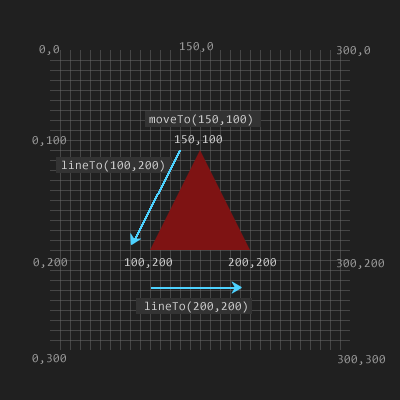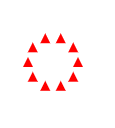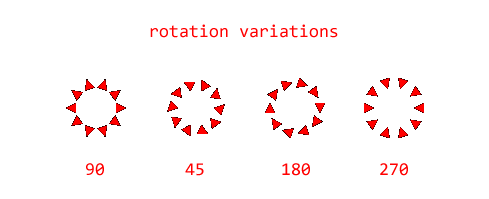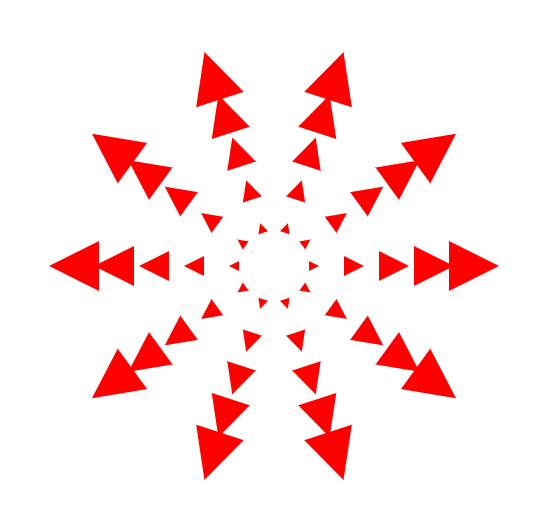## Canvas - Triangles

There’s no built in function in canvas for making triangles, but since a triangle is just a particular arrangement of 3 lines it should be easy enough to construct with lineTo:

``ctx.beginPath();ctx.moveTo(150, 100);ctx.lineTo(100, 200);ctx.lineTo(200, 200);ctx.fill();``When using fill(), open paths are closed automatically, from the current point back to the starting point. That’s why lineTo(150,100) wasn’t necessary at the end. If stroke() is used instead of fill, however, then a call to closePath() is required.

Rather than painstakingly arranging triangles at specific coordinates on the grid, a different approach is to move coordinates via translate and draw the triangle at 0,0. Using this approach to arrange a set of 10 triangles around the center of the canvas:

``ctx.clearRect(0, 0, w, h);ctx.fillStyle = "red";// Triangle pointsvar t = [];t = { x: 0, y: -5 };t = { x: -5, y: 5 };t = { x: 5, y: 5 };for (var deg = 0; deg < 360; deg += 36) {  var a = radians(deg);  var x = Math.cos(a) * 25;  var y = Math.sin(a) * 25;  ctx.translate(canvas.width / 2 + x, canvas.height / 2 + y);  drawTriangle(t);  ctx.setTransform(1, 0, 0, 1, 0, 0); //reset identity matrix}function drawTriangle(t) {  ctx.beginPath();  ctx.moveTo(t.x, t.y);  ctx.lineTo(t.x, t.y);  ctx.lineTo(t.x, t.y);  ctx.fill();}function radians(deg) {  return (deg * Math.PI) / 180;}``Now playing with the orientation of the triangles by adding the line:

``ctx.rotate(radians(deg + 90));``

just before the call to drawTriangle.Adding a scale function is a lazy way to draw multiple rings

``var p = [];p = { x: 0, y: -5 };p = { x: -5, y: 5 };p = { x: 5, y: 5 };ctx.clearRect(0, 0, w, h);ctx.fillStyle = "red";for (var i = 0; i < 4; i++) {  drawRing(p, 25, 36, 90, i);}function drawRing(p, r, stepBy, rDegrees, scale) {  r = r * scale + scale * 15;  for (var deg = 0; deg < 360; deg += stepBy) {    var a = radians(deg);    var x = Math.cos(a) * r;    var y = Math.sin(a) * r;    ctx.translate(w / 2 + x, h / 2 + y);    ctx.rotate(radians(deg + rDegrees));    ctx.scale(scale, scale);    drawTriangle(p);    ctx.setTransform(1, 0, 0, 1, 0, 0); //reset identity matrix  }}``Combining this exploration into a project:

See the Pen Triangle Spirals by Kentskyo (@kentskyo) on CodePen.